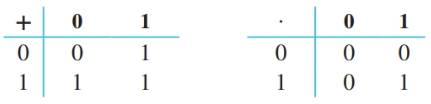Chapter 6.4, Problem 11ES### Discrete Mathematics With Applicat...

5th Edition
EPP + 1 other
ISBN: 9781337694193

#### Solutions

Chapter
Section### Discrete Mathematics With Applicat...

5th Edition
EPP + 1 other
ISBN: 9781337694193
Textbook Problem
1 views

# Let S = {O, 1}, and define operations + and · on S by the following tables:a. Show that the elements of S satisfy the following properties: (i) the commutative law for + (ii) the commutative law for ·(iii) the associative law for + (iv) the associative law for · (v) the distributive law for + over ·(vi) the distributive law for · over + b. Show that 0 is an identity element for + and that 1 is an identity element for ·. c. Define 0 ¯ = 1 and 1 ¯ = 0 . Show that for every a in S. a + a ¯ = 1 and a ⋅ a ¯ = 0 . It follows from parts (a)—(c) that S is a Boolean algebra with the operations + and ·.

To determine

(a)

To prove:

Show that the elements of S satisfies:

1. The commutative law for +
2. The commutative law for
3. The associative law for +
4. The associative law for
5. The distributive law for + over
6. The distributive law for over +
Explanation

Given information:

Let S={0,1}, and define operations + and on S by the following tables:

Proof:

(i) To proof: x+y=y+x

PROOF:

x+y=1 if at least one of x and y is 1.

x+y=0 if x and y are both 0.

First case: x = 0 and y = 0

x+y=0+0=0=0+0=y+x

Second case: x = 0 and y = 1

x+y=0+1=1=1+0=y+x

Third case: x = 1 and y = 0

x+y=1+0=1=0+1=y+x

Fourth case: x = 1 and y = 1

x+y=1+1=1=1+1=y+x

Thus, we note that the commutative law x+y=y+x is true in each case.

(ii) To proof: xy=yx

PROOF:

xy=1 if x and y are both 1.

xy=0 if at least one of x and y is 0.

First case: x = 0 and y =0

xy=00=0=00=yx

Second case: x = 0 and y = 1

xy=01=0=10=yx

Third case: x = 1 and y = 0

xy=10=0=01=yx

Fourth case: x = 1 and y = 1

xy=11=1=11=yx

Thus we note that the commutative law xy=yx is true in each case.

(iii) To proof: (x+y)+z=x+(y+z)

PROOF:

x+y=1 if at least one of x and y is 1.

x+y=0 if x and y are both 0.

First case: x = 0 and y = 0 and z = 0

(x+y)+z=(0+0)+0=0+0=0

x+(y+z)=0+(0+0)=0+0=0

Second case: x = 0 and y = 0 and z = 1

(x+y)+z=(0+0)+1=0+1=1

x+(y+z)=0+(0+1)=0+1=1

Third case: x = 0 and y = 1 and z = 0

(x+y)+z=(0+1)+0=1+0=1

x+(y+z)=0+(1+0)=0+1=1

Fourth case: x = 0 and y = 1 and z = 1

(x+y)+z=(0+1)+1=1+1=1

x+(y+z)=0+(1+1)=0+1=1

Fifth case: x = 1 and y = 0 and z = 0

(x+y)+z=(1+0)+0=1+0=1

x+(y+z)=1+(0+0)=1+0=1

Sixth case: x = 1 and y = 0 and z = 1

(x+y)+z=(1+0)+1=1+1=1

x+(y+z)=1+(0+1)=1+1=1

Seventh case: x = 1 and y = 1 and z = 0

(x+y)+z=(1+1)+0=1+0=1

x+(y+z)=1+(1+0)=1+1=1

Eight case: x = 1 and y = 1 and z = 1

(x+y)+z=(1+1)+1=1+1=1

x+(y+z)=1+(1+1)=1+1=1

Thus we note that the associative law (x+y)+z=x+(y+z) is true in each case.

(iv) To proof: (xy)z=x(yz)

PROOF:

xy=1 if x and y are both 1.

xy=0 if at least one of x and y is 0.

First case: x = 0 and y = 0 and z = 0

(xy)z=(00)0=00=0

x(yz)=0(00)=00=0

Second case: x = 0 and y = 1 and z = 0

(xy)z=(00)1=01=0

x(yz)=0(01)=00=0

Third case: x = 0 and y = 1 and z = 0

(xy)z=(01)0=00=0

x(yz)=0(10)=00=0

Fourth case: x = 0 and y = 1 and z = 1

(xy)z=(01)1=01=0

x(yz)=0(11)=01=0

Fifth case: x = 1 and y = 0 and z = 0

(xy)z=(10)0=00=0

x(yz)=1(00)=10=0

Sixth case: x = 1 and y = 0 and z = 1

(xy)z=(10)1=01=0

x(yz)=1(01)=10=0

Seventh case: x = 1 and y = 1 and z = 0

(xy)z=(11)0=10=0

x(yz)=1(10)=10=0

Eight case: x = 1 and y = 1 and z = 1

(xy)z=(11)1=11=1

x(yz)=1(11)=11=1

Thus we note that the associative law (xy)z=x(yz) is true in each case.

(v) To proof: x+(yz)=(x+y)(x+z)

PROOF:

x+y=1 if at least one of x and y is 1

To determine

(b)

To prove:

0 is an identity element for + and that 1 is an identity element for .

To determine

(c)

To prove:

For every a in S, a+a¯=1 and aa¯=0.

### Still sussing out bartleby?

Check out a sample textbook solution.

See a sample solution

#### The Solution to Your Study Problems

Bartleby provides explanations to thousands of textbook problems written by our experts, many with advanced degrees!

Get Started